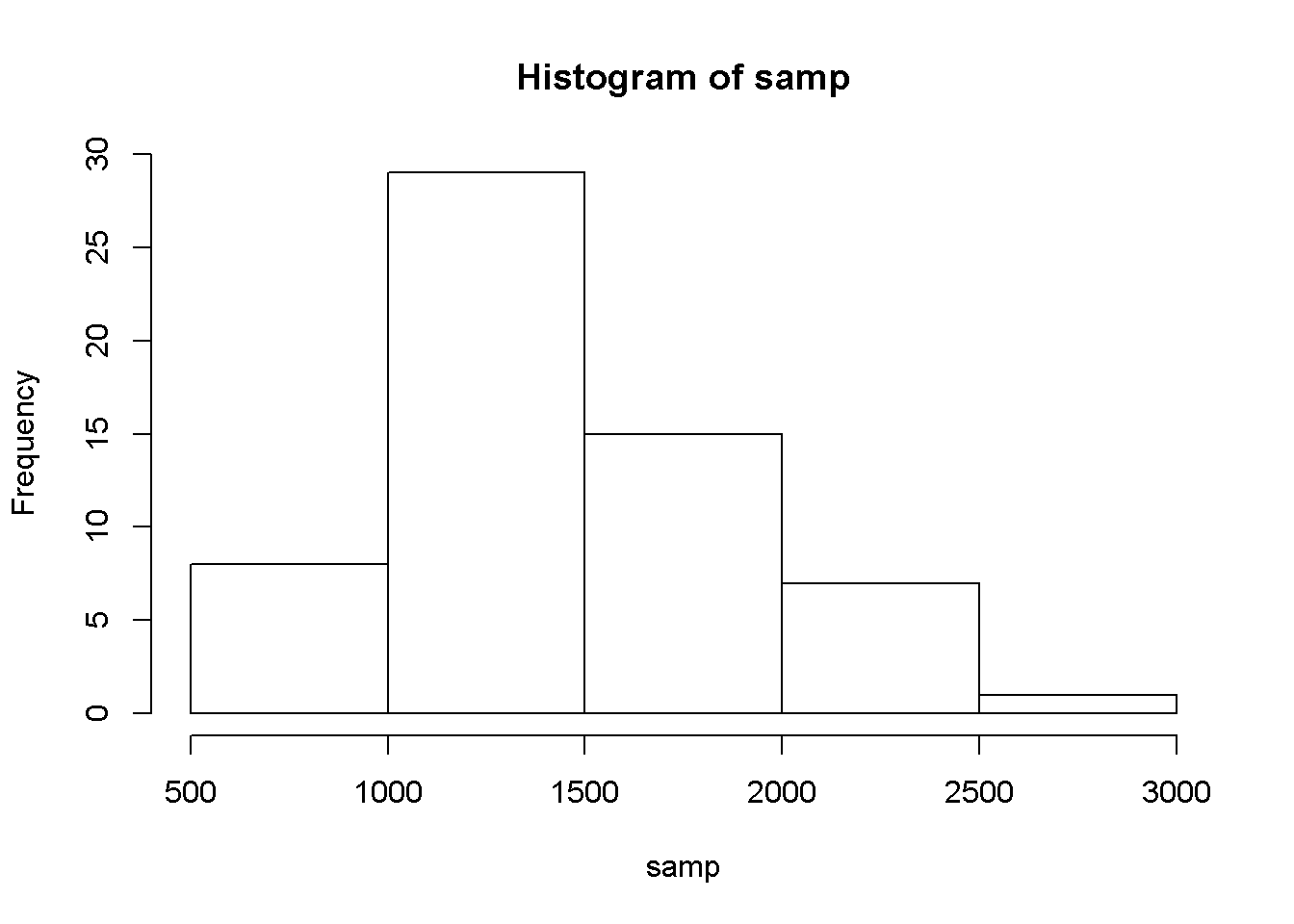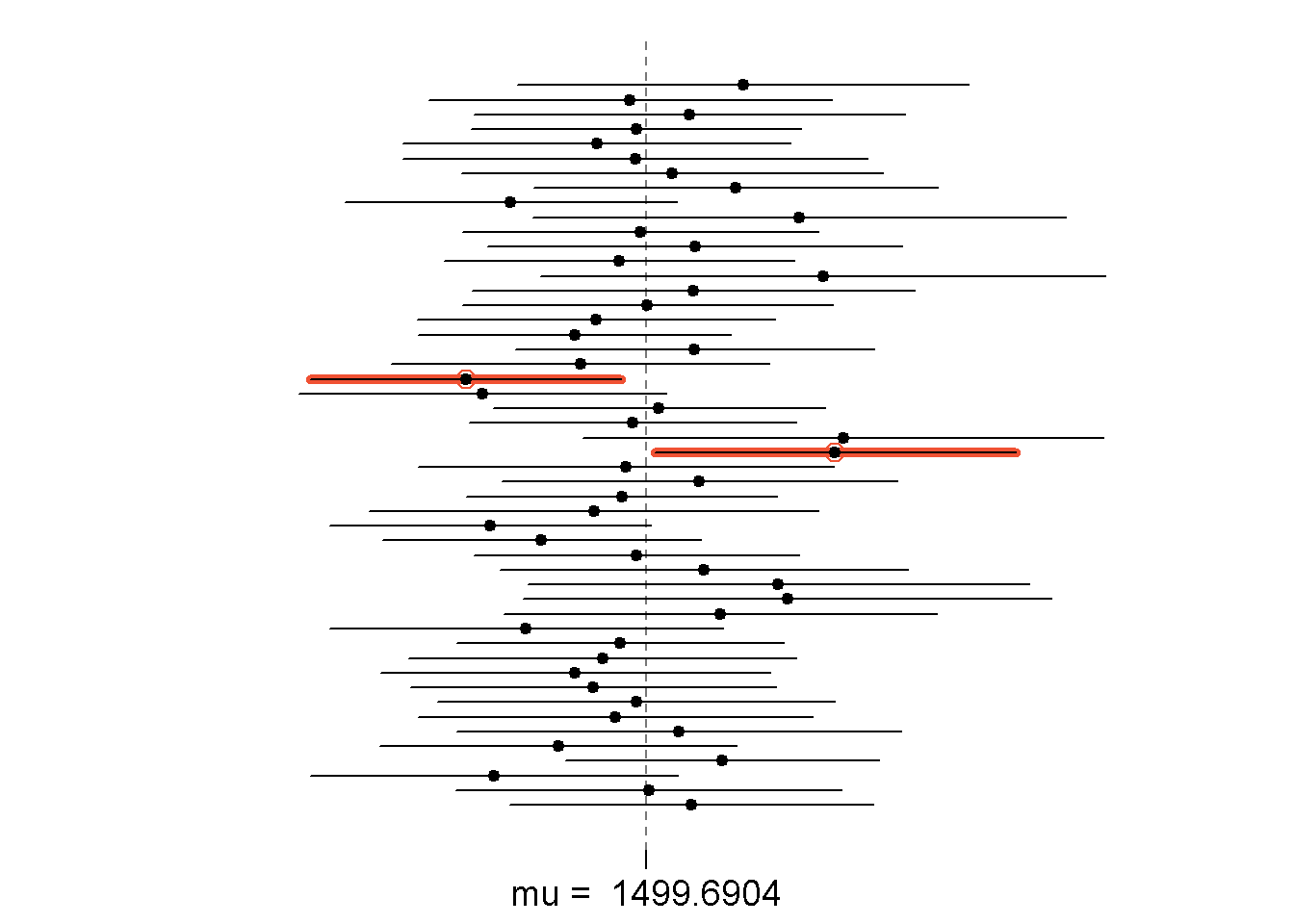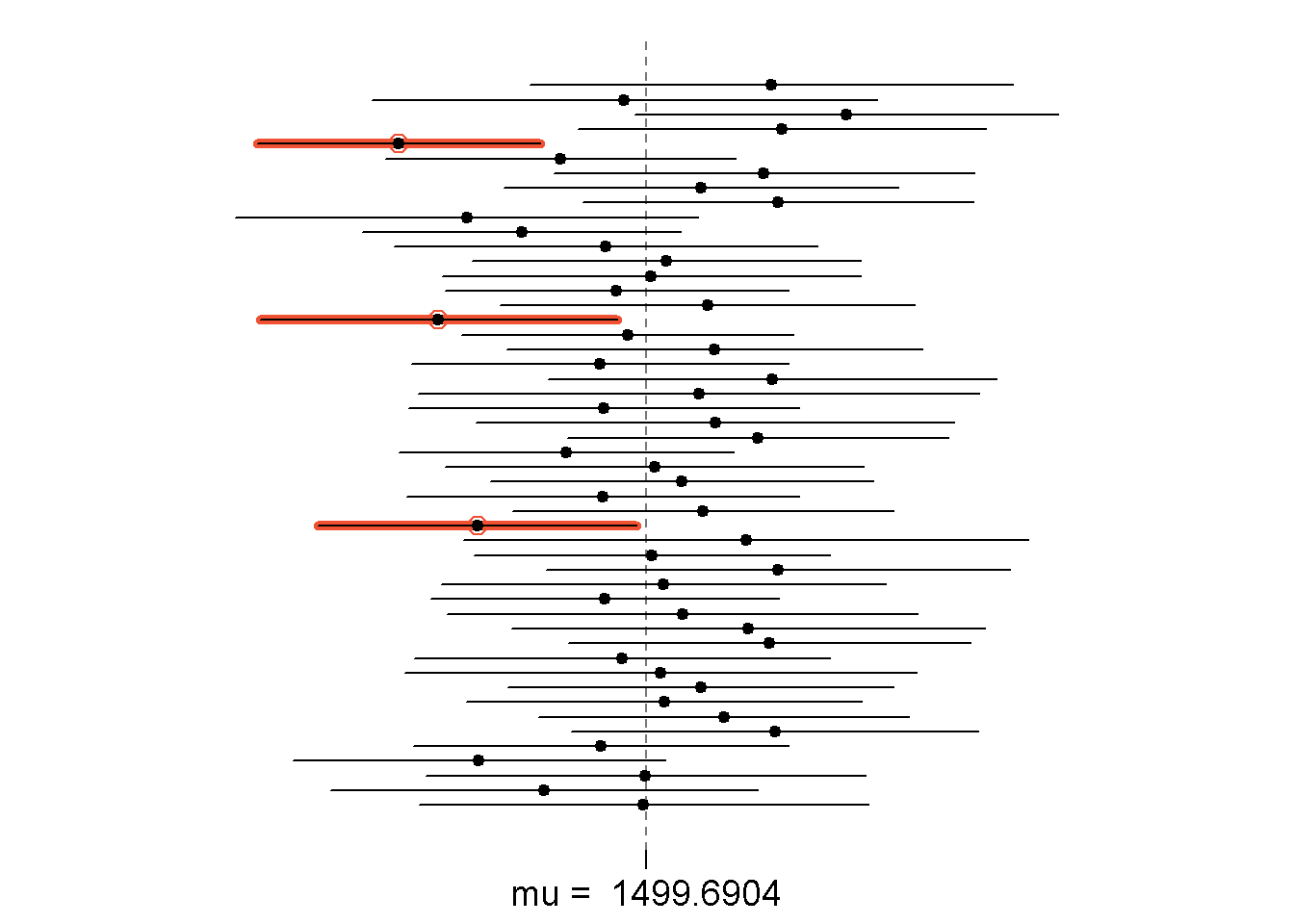## Sampling from Ames, Iowa

#### The Data

download.file("http://www.openintro.org/stat/data/ames.RData", destfile = "ames.RData")
load("ames.RData")
population <- ames\$Gr.Liv.Area
samp <- sample(population, 60)

### Exercise 1. Describe the distribution of your sample. What would you say is the “typical” size within your sample? Also state precisely what you interpreted “typical” to mean.

hist(samp)summary(samp)
##    Min. 1st Qu.  Median    Mean 3rd Qu.    Max.
##     616    1218    1368    1447    1628    2654

## Confidence Intervals

sample_mean <- mean(samp)
se <- sd(samp) / sqrt(60)
lower <- sample_mean - 1.96 * se
upper <- sample_mean + 1.96 * se
c(lower, upper)
##  1340.208 1553.425

## Confidence Levels

### Exercise 4. What does “95% confidence” mean?

#### 95% confidence means roughly 95% of the time the estimates that the true population mean is within two standard deviations around the sample mean.

mean(population)
##  1499.69

## Loop for 50samples

samp_mean <- rep(NA, 50)
samp_sd <- rep(NA, 50)
n <- 60
for(i in 1:50){
samp <- sample(population, n)
samp_mean[i] <- mean(samp)
samp_sd[i] <- sd(samp)
}
sd(samp)
##  576.9694
lower_vector <- samp_mean - 1.96 * samp_sd / sqrt(n)
upper_vector <- samp_mean + 1.96 * samp_sd / sqrt(n)
c(lower_vector, upper_vector)
##  1410.820 1646.413

### 1. Using the following function (which was downloaded with the data set), plot all intervals. What proportion of your confidence intervals include the true population mean? Is this proportion exactly equal to the confidence level? If not, explain why.

plot_ci(lower_vector, upper_vector, mean(population))#### 4 out of the 50 confidence interval did not include the true population mean of 1499.69, which is 92%. This proportion is not exactly equal to 95% confidence level because the confidence interval is a range to values that 95% of the estimate will contain the true population mean. ### 2. Pick a confidence level of your choosing, provided it is not 95%. What is the appropriate critical value?

qnorm(0.95,0,1)
##  1.644854

### 3.Calculate 50 confidence intervals at the confidence level you chose in the previous question. You do not need to obtain new samples, simply calculate new intervals based on the sample means and standard deviations you have already collected. Using the plot_ci function, plot all intervals and calculate the proportion of intervals that include the true population mean. How does this percentage compare to the confidence level selected for the intervals?

samp_mean2 <- rep(NA, 50)
samp_sd2 <- rep(NA, 50)
p <- 60
for(i in 1:50){
samp2 <- sample(population, p)
samp_mean2[i] <- mean(samp2)
samp_sd2[i] <- sd(samp2)
}
lower <- samp_mean2 - 1.645 * samp_sd2 / sqrt(p)
upper <- samp_mean2 + 1.645 * samp_sd2 / sqrt(p)
c(lower, upper)
##  1377.575 1618.559
plot_ci(lower, upper, mean(population))